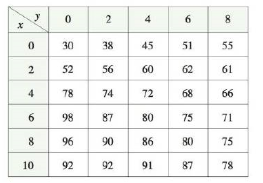Chapter 14, Problem 11RE

Chapter
Section
Textbook Problem

A metal plate is situated in the xy-plane and occupies the rectangle 0 ≤ x 10, 0 ≤ y ≤ 8, where x and y are measured in meters. The temperature at the point (x, y) in the plate is T(x, y), where T is measured in degrees Celsius. Temperatures at equally spaced points were measured and recorded in the table.(a) Estimate the values of the partial derivatives Tx(6, 4) and Ty(6,4). What arc the units?(b) Estimate the value of DuT(6, 4), where u = ( i + j ) / 2 .(c) Estimate the value of Txy(6,4).(a)

To determine

To estimate: The value of the partial derivatives Tx(x,y)andTy(x,y) at (6,4) and its unit.

Explanation

Given:

The function is, T(x,y) .

Where T is temperature and measured in degree Celsius.

xandy are measured in meters.

Calculation:

Find the value of Tx(6,4) as follows,

Tx(6,4)=limh0T(6+h,4)T(6,4)h

Let h=2 and find the value Tx(6,4) ,

Tx(6,4)=limh0T(6+2,4)T(6,4)2=limh0T(8,4)T(6,4)2=86802=3

Let h=2 and find the value Tx(6,4) ,

Tx(6,4)=limh0T(62,4)T(6,4)2=limh0T(4,4)T(6,4)2=72802=4

Compute the average value of Tx(6,4)=3 and Tx(6,4)=4 .

Tx(6,4)=3+42=72=3.5

Thus, the value of Tx(6,4)=3

(b)

To determine

To estimate: The directional derivative of T(x,y) at the point (6,4) in the direction unit vector u=(i+j)2 and interpret it.

(c)

To determine

To estimate: The value of Txy(x,y) at the point (6,4) .

Still sussing out bartleby?

Check out a sample textbook solution.

See a sample solution

The Solution to Your Study Problems

Bartleby provides explanations to thousands of textbook problems written by our experts, many with advanced degrees!

Get Started

A population of N = 7 scores has a mean of = 13. What is the value of X for this population?

Essentials of Statistics for The Behavioral Sciences (MindTap Course List)

In Exercises 79-84,determine whether the statement is true or false for all real numbers a and b. 79. |a| = a

Applied Calculus for the Managerial, Life, and Social Sciences: A Brief Approach

Solve the equation in Problem 1-6. 4.

Mathematical Applications for the Management, Life, and Social Sciences

Find the slope of each line: y=5x7

Elementary Technical Mathematics